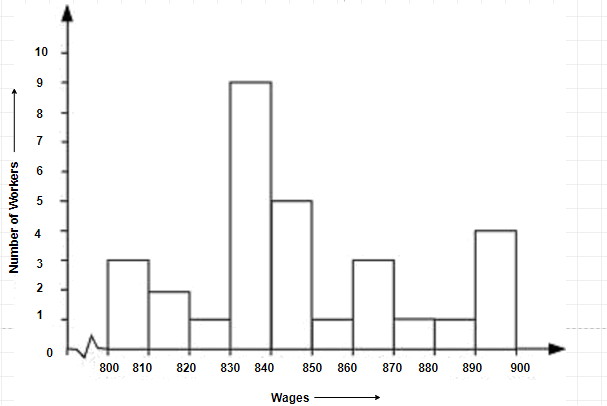# Ex.5.1 Q4 Data Handling Solutions - NCERT Maths Class 8

## Question

Draw a histogram for the frequency table made for the data in Question $$3$$ and answer the following questions.

(i) How many workers earn $$\rm{Rs}\; 850$$ and more?

(ii) How many workers earn less than $$\rm{Rs}\;850$$?

Video Solution
Data Handling
Ex 5.1 | Question 4

## Text Solution

What is known?

Weekly wages of $$30$$ workers in Rupees.

What is unknown?

1.  Number of workers earning $$850$$ or more.

2.  Number of workers earning less than $$850.$$

Reasoning:

Number of occurrences of the particular entry is known as frequency.

Steps:

(i) $$830\,– \,840$$ group has the maximum number of workers.

(ii) $$10$$ workers can earn more than $$\rm{Rs}\;850.$$

(iii) $$20$$ workers earn less than $$\rm{Rs}\;850.$$Learn from the best math teachers and top your exams

• Live one on one classroom and doubt clearing
• Practice worksheets in and after class for conceptual clarity
• Personalized curriculum to keep up with school